## Table of ContentOpen Access

ARTICLE

# Classification of Nonlinear Confusion Component Using Hybrid Multi-Criteria Decision Making

Nabilah Abughazalah1, Iqra Ishaque2, Majid Khan2,*, Ammar S. Alanazi3, Iqtadar Hussain4,5

1 Department of Mathematical Sciences, College of Science, Princess Nourah bint Abdulrahman University, P.O.Box 84428, Riyadh 11671, Saudi Arabia
2 Department of Applied Mathematics and Statistics, Institute of Space Technology, Islamabad, Pakistan
3 Department of Mathematics, King Abdulaziz University, Faculty of Science, Jeddah, Saudi Arabia
4 Mathematics Program, Department of Mathematics, Statistics and Physics, College of Arts and Sciences, Qatar University, 2713, Doha, Qatar
5 Statistical Consulting Unit, College of Arts and Science, Qatar University, Doha, Qatar

* Corresponding Author: Majid Khan. Email:Intelligent Automation & Soft Computing 2023, 36(2), 1451-1463. https://doi.org/10.32604/iasc.2023.031855

## Abstract

In today’s digital world, the most inevitable challenge is the protection of digital information. Due to the weak confidentiality preserving techniques, the existing world is facing several digital information breaches. To make our digital data indecipherable to the unauthorized person, a technique for finding a cryptographically strong Substitution box (S-box) have presented. An S-box with sound cryptographic assets such as nonlinearity (NL), strict avalanche criterion (SAC), bit independence criteria (BIC), bit independence criteria of nonlinearity (BIC-NL), Bit independence criteria of Strict avalanche criteria (BIC-SAC), and Input/output XOR is considered as the robust S-box. The Decision-Making Trial and Evaluation Laboratory (DEMATEL) approach of multi-criteria decision making (MCDM) is proposed for finding the interrelation among cryptographic properties. A combination of two MCDM methods namely Entropy and multi-objective optimization based on ratio analysis (MOORA) is applied for the best S-box selection. A robust substitution box is selected for secure communications in cryptography by using the combination of DEMETAL selection criteria, entropy weight assigning, and MOORA ranking scheme. The combination of these three methods provides a fast selection procedure for the secure confusion component. The offered selection method can also be utilized for the choice of the best cryptosystem with highly secure properties and resistive against all possible linear and differential attacks in the cryptanalysis.

## Keywords

1  Introduction

Communication over public channels is becoming increasingly common, implying that approved access is required. The rapid development of multimedia technology, as well as digital content such as photographs, video, and audio, has a significant impact on communication. Since information is conveyed from one end to the other in these advanced communication methods . As a result, the protection of this digital data is an unavoidable concern. To meet the privacy requirements of such contents, appropriate protection tools must be established . Classified information can be kept confidential using a variety of security measures. These systems used encryption, which is the method of converting original data into an unreadable format . An S-box is a crucial tool, and it is widely used in the field of cryptography . The S-box is the only non-linear component in an encryption system that provides necessary confusion. The development of powerful encryption systems necessitates the structure of S-boxes with perfect cryptographic properties. Chaotic S-boxes based on time-delay chaotic systems have been proposed by Yuvaz et al. in . In block ciphers, substitution boxes with robust cryptographic properties are commonly used to provide the important property of nonlinearity. They’re necessary to fend off common attacks like linear and differential cryptanalysis . Hussain et al., assembled S-boxes using an algorithm based on linear fractional transform .

Effective decision-making is becoming more desirable as the environment becomes more complex . Decision-makers must always evaluate a dynamic and perplexing situation, determine the cause of a problem, choose an acceptable solution, and implement an effective action plan . Their success is primarily determined by their ability to think objectively about the causal relationship .

Multi-criteria decision-making (MCDM) methods offer decision-makers a variety of tools and enable them to decide between multiple conflicting criteria . MCDM methods to real-world decisions, the advancement in technology over the last few decades have allowed for the development of more sophisticated decision analysis methods. There are several approaches available for effective decision-making. Each approach employs numerical techniques to assist decision-makers in selecting from a selection of discrete alternatives . This is accomplished by evaluating the effect of the alternatives on specific parameters and, as a result, the decision maker’s overall usefulness .

The proposed approach used the DEMATEL method to apply an MCDM model . This method identifies the most suitable alternative in terms of the observed criteria and then compares it to the optimal solution by calculating the distances between other options based on the observed ideal value criterion. The foremost attribute of the DEMATEL method is constructing interrelations among criteria. To discriminate among cause-and-effect groups among different criteria this method provides a way .

Fig. 1 describes a list of several commonly used multi-criteria decision-making approaches to solving different multiple criteria problems in the real world: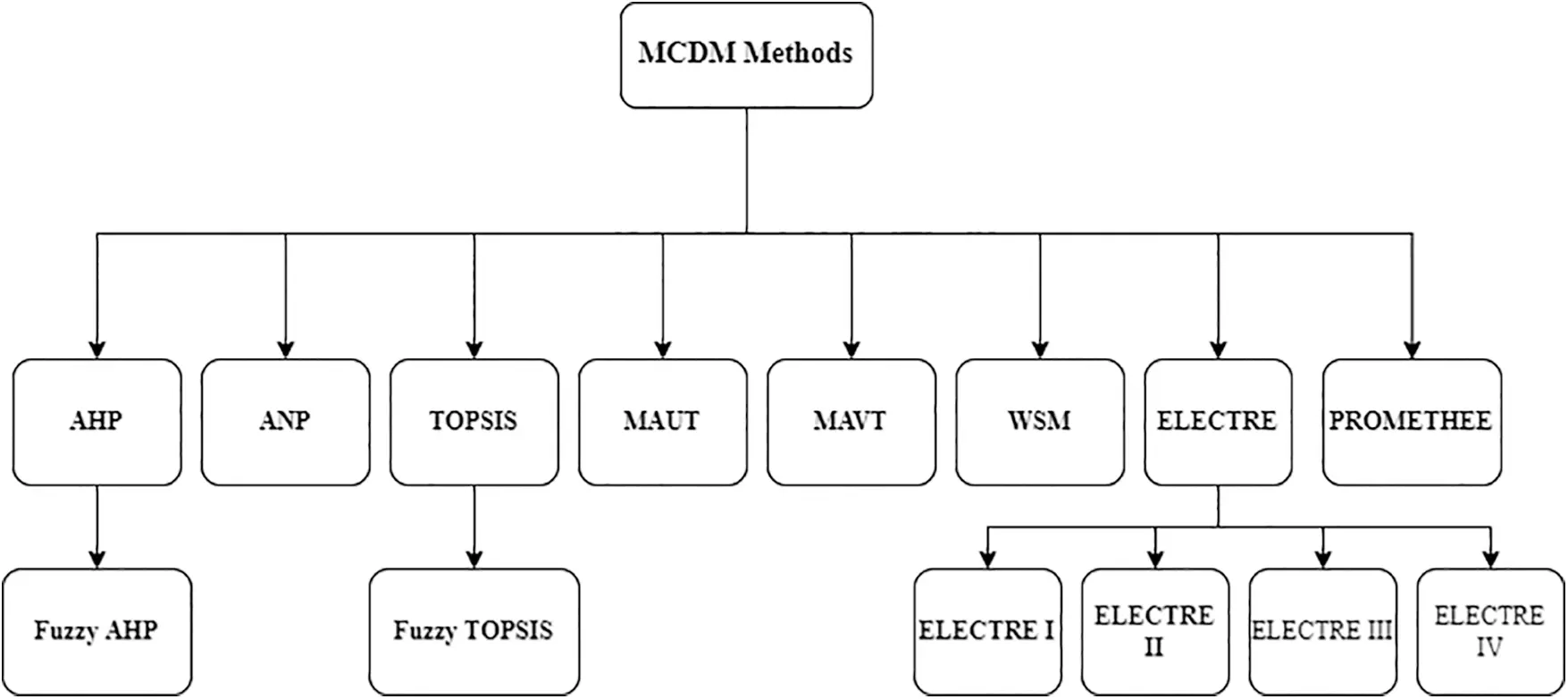Figure 1: Flow chart of some common MCDM approaches

In the present work, an MCDM technique has been employed for finding the best S-box . We have considered some standard S-boxes and a proposed S-box which include AES, Skipjack, Xyi, Residue Prime, and Model 1. The algebraic properties of these S-boxes which we have considered are, Nonlinearity, Strict Avalanche Criteria (SAC), Bit Independence Criteria for Nonlinearity (BIC-NL), Bit Independence Criteria Strict Avalanche Criteria (BIC-SAC), Input/output XOR [23,24]. The Decision-Making Trial and Evaluation Laboratory (DEMATEL) method is used, which is a sort of structural modeling approach, that can separate the involved criteria of a system into the cause group and effect group. Entropy is an objective weighting assigning technique used to assign weights to the criteria and S-boxes are ranked using the MOORA method [25,26]. In any encryption system, S-box is responsible for providing necessary confusion, so we will employ MCDM methods to get the robust S-box.

Research Objectives

The main objectives of this research are as follows:

1.    To offer a fast selection method using the combination of DEMATEL, entropy, and MOORA methods for decision making, weight assigning, and alternative ranking respectively.

2.    To utilize the suggested method for the best selection of confusion components.

3.    To increase the speed of selection and choose the best alternative in the minimum time.

4.    To get the S-box with high-nonlinearity and other ideal properties.

5.    To form a standard method to achieve the ideal selection criteria for other components and cryptosystems in cryptography.

The rest of the manuscript is arranged as follows: Section 2 describes some basic assets related to the substitution box, Section 3 includes the basics and mathematical formulation of the DEMATEL method, the entropy method for weight assigning is defined in Section 4, and MOORA scheme for ranking is depicted in Section 5, conclusion and future recommendations are presented in the last section.

2  Preliminaries

In this segment, we will add some fundamental characteristics of nonlinear confusion components. Our optimum selection of robust nonlinear confusion components is based on these standards and mostly utilized cryptographic properties .

2.1 Nonlinearity

Let Nh be a boolean function then nonlinearity can be defined as its lowest possible distance to any affine function. Nonlinearity is calculated as:

Nh=12(2nWHTmax(h)),

where WHTmax(h) represents the Walsh-Hadamard transformation of a Boolean function defined as:

F^h(α)=xBnh^(x)L^α(x),

where, h^(x)=(1)h(x) is associated characteristic function with Boolean function.

2.2 Strict Avalanche Criteria (SAC)

This criterion necessities that for an S-box employed in an encryption scheme if any single information bit i is reversed then there exist a likelihood of 50% that the output bit j is altered i,j . This criterion expands the property of completeness and redefines the criteria of the avalanche. An S-box is said to satisfy the Strict Avalanche Criterion (SAC) if half of its output bit alters whenever there is a variation in an individual input bit. For strict avalanche criteria, an optimum value is 0.5.

2.3 Bit Independence Criteria for Nonlinearity (BIC-NL)

When a single input bit changes for all i, j, and k output bits j, and k must be updated. A highly non-linear Boolean function for two output bits is required to ensure that the correlation between them is zero, as Adam and Tavares pointed out when an input bit was inverted.

2.4 Bit Independence Criteria of Strict Avalanche Criteria (BIC-SAC)

This criterion requires avalanche variables to be pairwise independent. It signifies that for a specified set of avalanche vectors generated, upon completing just one bit the avalanche variables must be pairwise independent.

2.5 Input/Output XOR or Differential Uniformity

Input variations can be used to generate output variations, and each output’s XOR value must have a similar possibility as the XOR value of every input. It means that an S-box is resilient to differential cryptanalysis if the input/output probability distribution is closed. To provide sensible protection against differential attacks, S-box must have a small value of differential uniformity Ω .

The details of all these cryptographic characteristics for benchmark nonlinear components are given in Tab. 1. The selection of the best confusion component founded on the given six cryptographic criteria is the aim of our article.3  Decision Making Trial and Evaluation Laboratory (DEMATEL) Technique

The DEMATEL procedure was first proposed by the Science and Human Affairs Program of the Battle Memorial Institute of Geneva from 1972 to 1976. This method aims at identifying the relationship between cause and effect between certain selected criteria. This approach is comprehensive in the analysis and construction of models which are related to each other. The method seeks to find instantaneous or immediate relations (dependence) between variables in a system. It may also approve interdependence between the components and create a map that represents the links between them to address complex decision-making difficulties. DEMATEL can divide interdependency relationships into two groups: cause and effect. In a complicated structural system, it may also figure out the key factors with the use of the influential relation map .

Mathematical Formulation of the DEMATEL Method

The DEMATEL approach assumes that a system has a collection of components with evaluable pair-wise relationships. Let B={B1,B2,B3,,Bn} be the components whose pair-wise relations can be evaluated and let {ζ1:NL, ζ2:SAC, ζ3:BICSAC, ζ4:BICNL, ζ1:I/O  XOR} be the set of criteria. This method exhibits dependency among attributes and limits the relationship that considers the assets with an important structure and improvement pattern based on the properties of objective affairs. The DEMATEL method produces a graphical interpretation of its final product .

Step 1: Formulation of Decision matrix.

Step 2: Obtaining direct relation matrix requires a rating scale; each criterion is rated by the decision-maker using the scale given in Tab. 2. The sum of these values is calculated using Eq. (1):

j=1naik (1)

Step 3: Direct relation matrix is normalized utilizing Eqs. (2) and (3):

X=kA, (2)

k=1max1ink=1naiki,k=1,2,,n (3)

Step 4: Let X={x1,x2,x3,,xn} be the normalized decision matrix then using Eq. (4) the total relation matrix can be obtained which gives the measure of how one factor or criteria affects the other:

T=X(1X)1. (4)

Step 5: E+R and ER are calculated, a greater magnitude of E+R shows that criteria have maximum relation with other criteria, and its lesser magnitude shows minimum relationship with other criteria. A criterion having the highest value of E+R is the most important criterion. A positive value of E−R signifies it belongs to the cause group also known as dispatcher. These criteria influence other criteria. A Negative value of E−R signifies that it belongs to the receiver group; they fall under the effect group which means these criteria get affected by other criteria.

Step 6: Construction of Causal diagram.

Based on these steps, the DEMATEL method is based on the subsequent strides:

Step 1: Defining the decision matrixTable 2: Contrast range of the DEMATEL procedure

Step: 2 Formulation of the direct relation matrix is described in Tab. 3.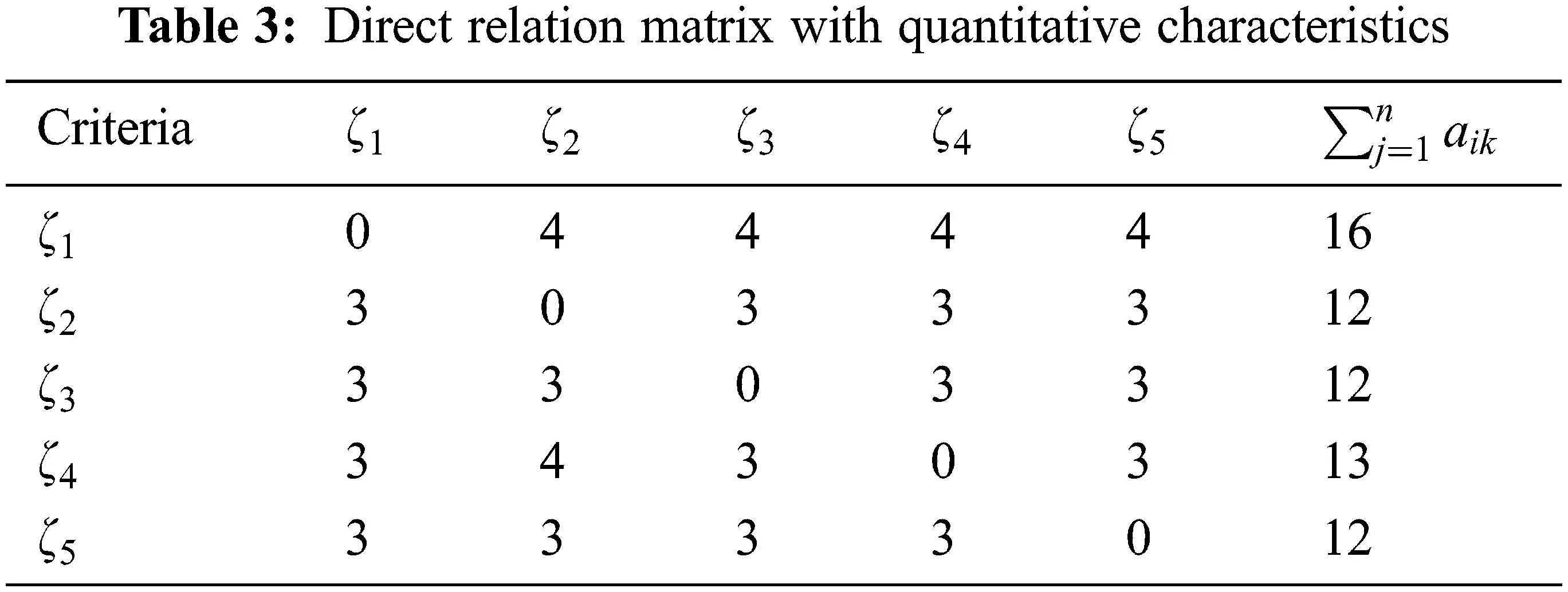Step: 3 In this step the decision matrix is normalized utilizing Eq. (5). Tab. 4 describes the normalized direct relation matrix.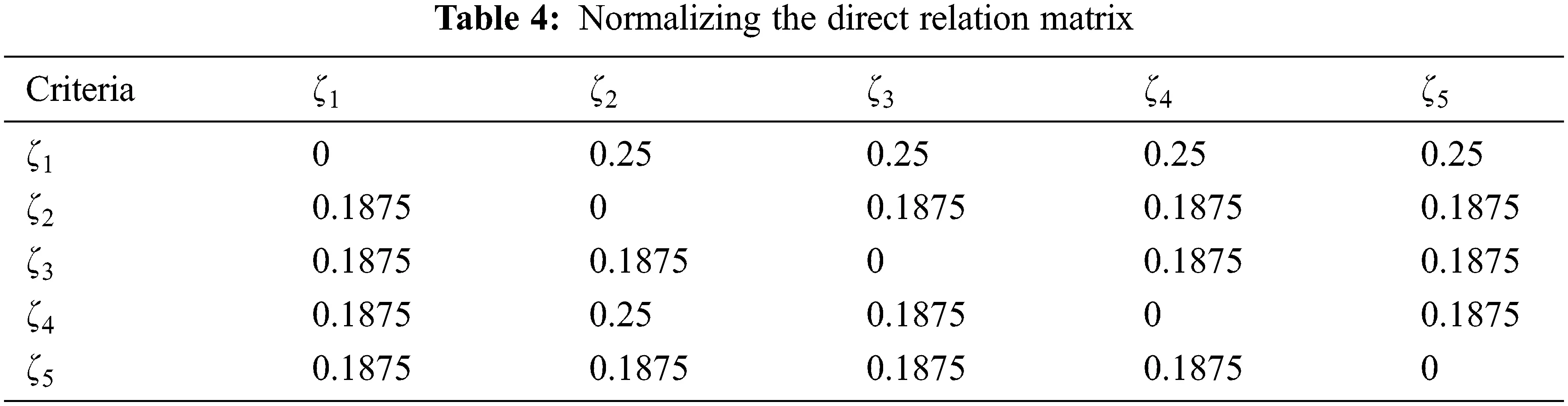X=k.A

k=1max1ink=1naik,i,k=1,2,.,n (5)

Step 4: In this phase, the total relation matrix is computed. The total relation matrix gives the measure of how one factor or criteria affects the other. Let X={x1,x2,x3,,xn} be the normalized decision matrix then Eq. (6) is employed in calculating the total relation matrix T (Tab. 5)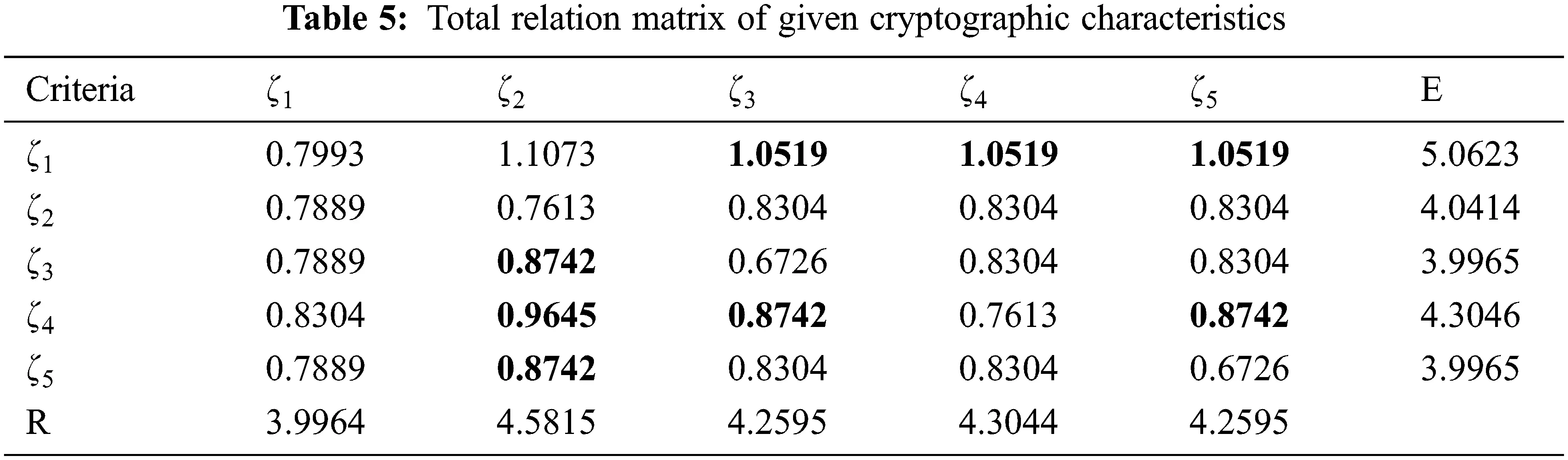T=X(1X)1 (6)

Step 5: In this step rows and columns of the total relation matrix are summed up which have been presented in Tab. 6.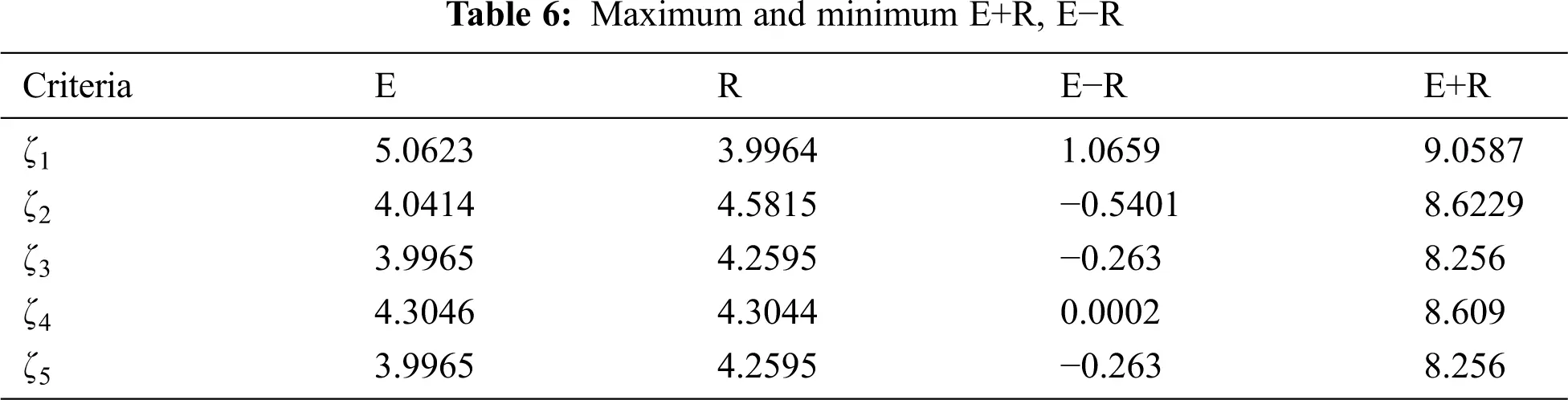E+R values:

A criterion having a greater value of E+R has the maximum relationship with other criteria, and those having a lesser value of E+R have a lesser relationship with other criteria. A criterion having the highest value of E+R is the most important criterion. We can see in Tab. 6 that nonlinearity has the highest E+R value of 9.0587 which indicates that nonlinearity is the most important criterion.

E−R values:

E−R tells the kind of relation among criteria. A positive value of E−R denotes it belongs to the cause group also known as dispatcher. These criteria influence other criteria. A Negative value of E−R indicates it belongs to the receiver group; they fall under the effect group which means these criteria get affected by other criteria. We can see in Tab. 6 that NL and BIC-NL fall under the cause group, whereas SAC, BIC-SAC, and Input/output XOR fall under the effect group.

Step 6: Constructing a causal diagram

The graphical relationship has been constructed in Fig. 2. The criteria involved are Non-linearity, SAC, BIC of SAC, BIC of Non-linearity, and Input/output XOR. In this step, an average value of all the criteria presented in Tab. 7 is calculated termed the threshold value. In our case, the threshold value is α = 0.856052 (Fig. 3).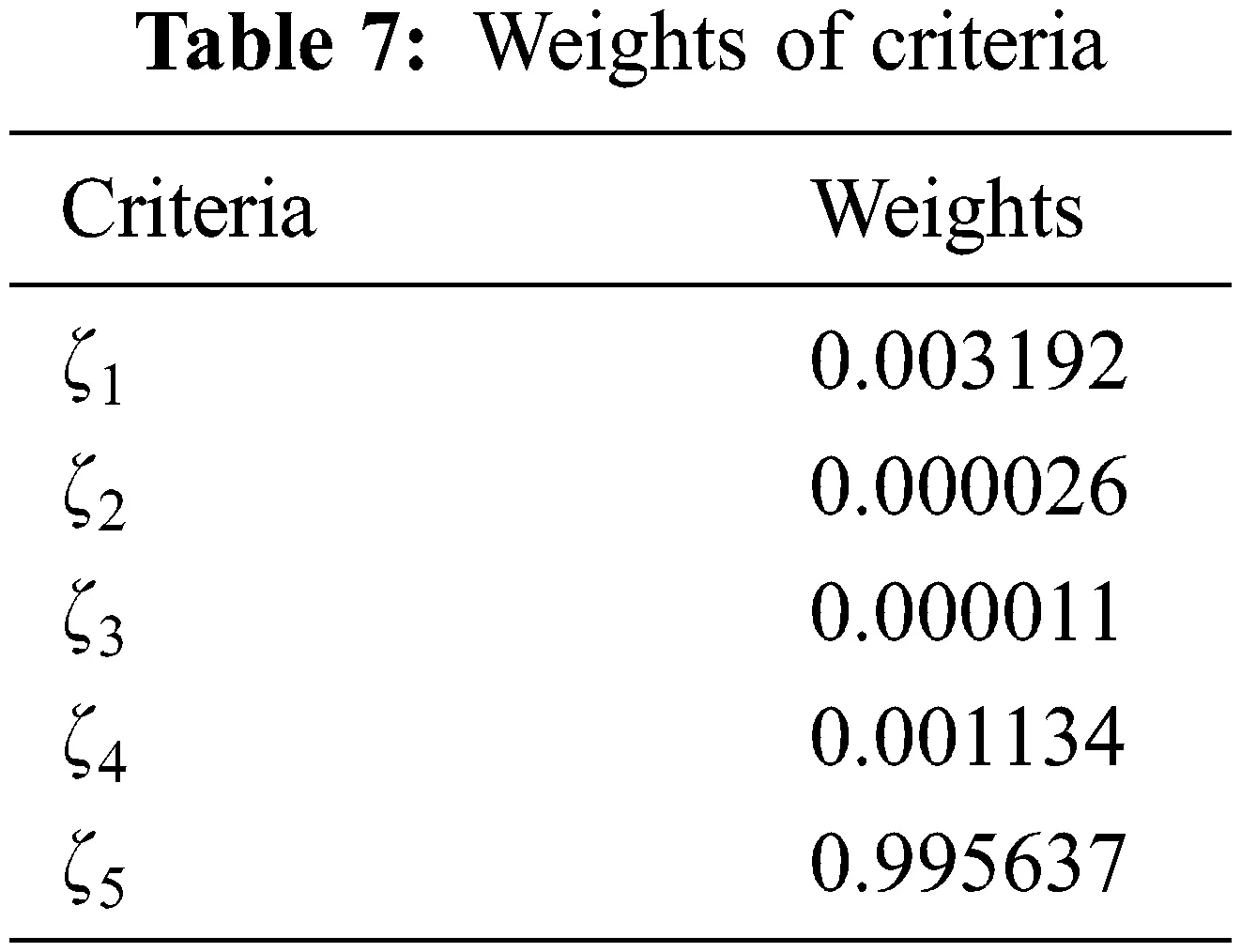It can be seen in Fig. 2 that non-linearity has a maximum relationship with Bit independence criteria of strict avalanche criteria, input/output XOR, and Bit independence criteria of non-linearity. Bit independence criteria of non-linearity have a relationship with SAC and BIC of SAC. Similarly, Bit independence criteria of strict avalanche criteria have a relationship with strict avalanche criteria. Input/output XOR has its relationship with Strict avalanche criteria.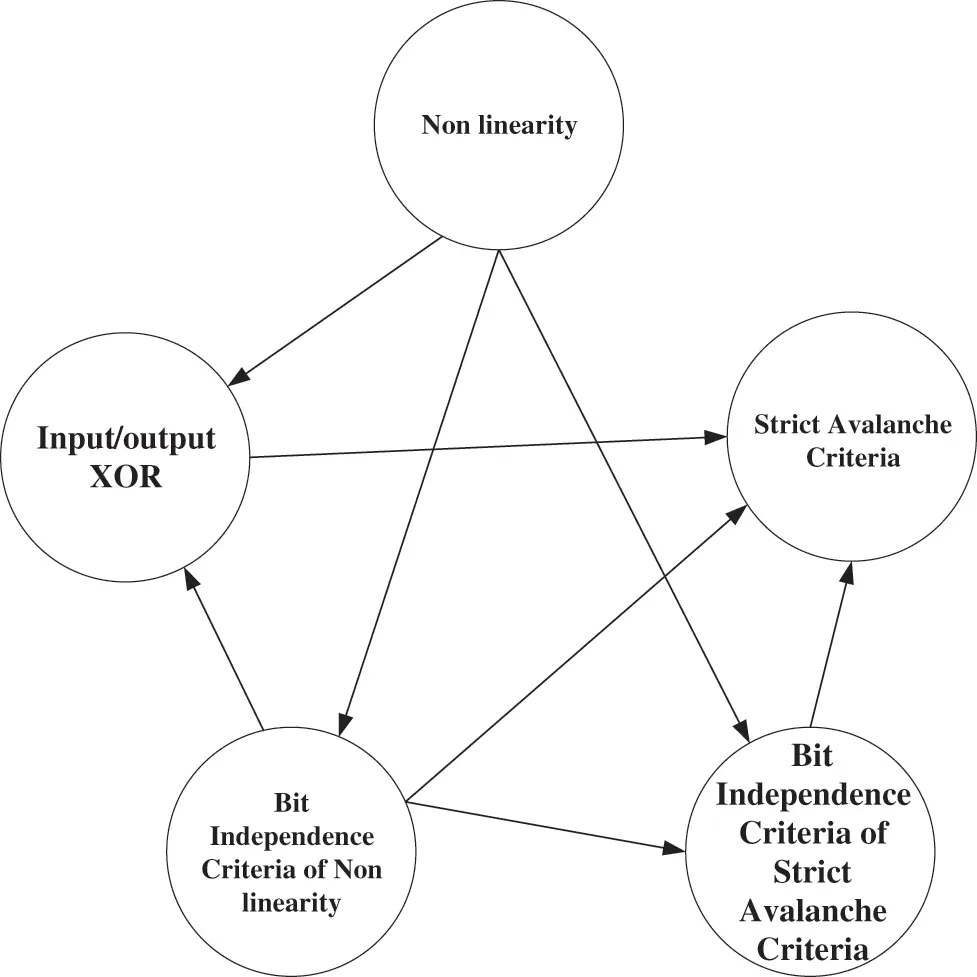Figure 2: Causal diagram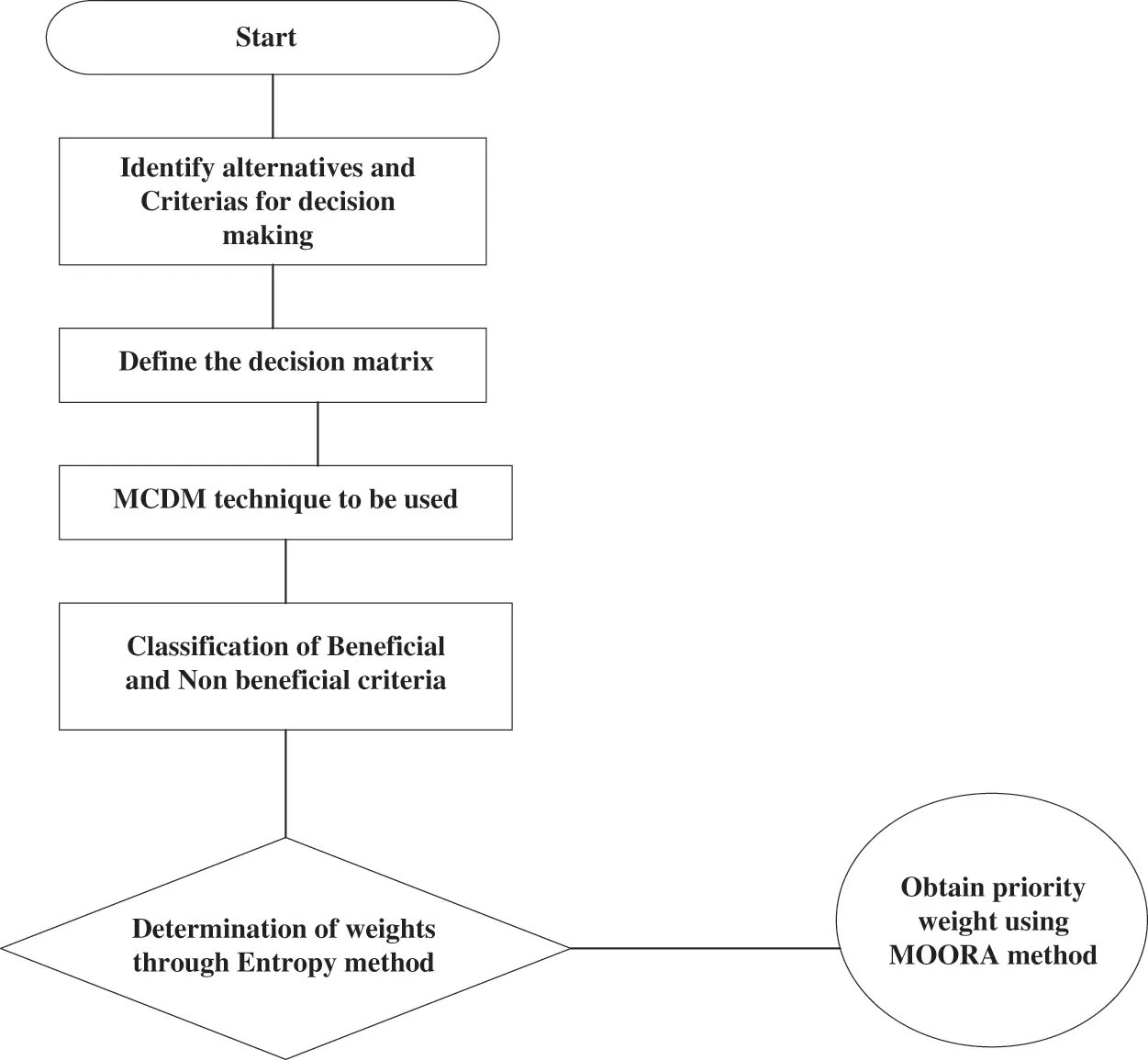Figure 3: Implementation of the proposed combination of Entropy and MOORA to determine the most vital criteria for the best confusion component of modern block ciphers

4  Entropy Method for Assigning Weights

The entropy method has been formulated using probability theory; it measures uncertainty in the given information. Using the entropy approach, one may analyze a predetermined decision matrix. A wide distribution transmits more uncertainty than a tightly packed one, according to entropy in information theory, criteria for the amount of uncertainty represented by a discrete probability distribution . The more the degree of dispersion, the more prominent the level of separation, and more data can be inferred. The greatest benefit of the entropy weighting method (EWM) is the aversion to human components obstructing the weights of indicators, thereby improving the objectivity of the overall assessment outcomes .

The Mathematical Formulation of the Entropy Method

The major objective of this part of the study is to discover the most significant cryptographic character of the S-box and its classification. In the present subsection, we are now adding a mathematical formulation of the Entropy method. This technique is based on probability theory which calculates uncertainty among given information.

Step: 1 In the first step the decision matrix is normalized. Let X={x1,x2,x3,,xn} be the decision matrix, to calculate weights by entropy approach the information matrix is normalized using the mathematical relation:

sik=xiki=1mxik (7)

Step: 2 This step calculates entropy value using the Eq. (8):

ek=ki=1msiklnsik,k=1,2,3,,n (8)

Step: 3 In this step the weight assigning vector wj is computed for each criterion. It is based upon the sum k=1n1ek and is divided by 1ek . The weight vector wk is given by:

wk=1ekk=1n1ek,k=1,2,3,,n (9)

On this basis step entropy structure comprises the subsequent strides:

Step 1: Normalizing the decision matrix

Let X={x1,x2,x3,,xn} be the decision matrix, to determine the weights by entropy process first the information matrix is normalized using the mathematical relation described in Eq. (7):

sik=xiki=1mxik,

where xik is the original measured data after obtaining the normalized matrix.

Step 2: Calculating the entropy value

The value of entropy can be assessed using the subsequent mathematical structure:

ek=ki=1msiklnsik,k=1,2,3,,n,

where k=1lnp and ‘ p ’ denotes the total number of choices. By taking p=5 , k=1ln(5)=0.6213 .

Step 3: Calculating weight vector

The weight vector wk assigns weight to each criterion, the sum k=1n1ek is divided by 1ek . The following mathematical relation provides the weight vector wk :

wk=1ekk=1n1ek,k=1,2,3,..,n

5  Multi-Objective Optimization Based on Ratio Analysis (MOORA) Method for Ranking

MOORA technique, first presented by Brauers et al. , is a multi-target improvement procedure that applies to any sort of complex decision-related issues. The MOORA technique consists of an initial decision matrix that contains the performance of different alternatives by taking into consideration various attributes.

The Mathematical Formulation of the MOORA Scheme

Step: 1 This method starts with a decision matrix that has m alternatives and n attributes

[x11x1nxm1xmn]

where xik is the execution of ith alternative on kth the attribute. The MOORA approach uses a ratio system to compare an alternative’s answer to a denominator, which is the representative for all alternatives related to that aim.

xik=xik[i=1mxik2]12,k=1,2,3,,n (10)

Step: 2 This step is known as optimization. In optimization, for the case of maximization responses are added and for minimization, responses are subtracted. This step is done using the following mathematical relation:

yi=k=1gxikk=g+1nxik (11)

Step: 3 In this step weights wk of kth obtained by entropy method are multiplied with xik , yi ’s are calculated using the following mathematical relation:

yi=k=1gwkxikk=g+1nwkxik (12)

The sum of the decision matrix’s maxima (benefit qualities) and minima (negative attributes) determines whether the yi values are positive or negative (non-beneficial attributes). To express one’s preference, one must provide an ordinal ordering of yi . Thus, the best choice has the highest value, while the worst alternative has the lowest value consequently.

The yi values can attain a positive or negative value depending on the sum of the decision matrix’s maxima (beneficial attributes) and minima (non-beneficial attributes). Preferences are made by showing an ordinal ranking of yi . As a result, the best option has the highest value, while the worst option has the lowest.

Multi-Objective optimizations based on Ratio Analysis (MOORA) method consist of the following steps:

Step: 1 Normalization

Let [x11x1nxm1xmn] be the decision matrix where xij is the performance of ith alternative on kth attribute, with m alternatives and n attributes. This is followed by the development of a ratio system, in which the accomplishments of each individual on a given attribute are measured against a denominator representing all of the possible outcomes for that attribute. It was discovered by Brauers et al. that the root of the sum of squares of each alternative for each characteristic is the sole possibility for this denominator when using various ratio systems, which include total ratios like Schärlig and Weitendorf as well as Jüttler and Stopp and Körth . The ratio has been presented in Eq. (10).

Step: 2 Optimization

In this step in case of maximization, responses are added and subtracted otherwise. This step is done using the mathematical relation given in Eq. (11).

Step: 3 Multiplying weights

In this step weights wk of kth obtained by the entropy method are multiplied with xik , yi ’s are calculated using the mathematical relation given in Eq. (12).

Tab. 8 represents the ranking of the alternatives using the MOORA method. It can be seen that the S-box of AES is ranked first with higher values of nonlinearity, BIC nonlinearity, SAC, and BIC-SAC, whereas the lowest value of I/O XOR. This shows that the S-box of AES is the best choice to be considered in an encryption scheme to achieve confusion.6  Conclusion and Future Recommendations

In this paper, we aimed at finding the robust S-box with sound algebraic properties such as nonlinearity, SAC, BIC, BIC-SAC, BIC-NL, and DU. Multi-criteria decision-making method DEMATEL has been employed to study the interrelation among criteria which shows that Nonlinearity has the most impact on other criteria, which makes it the most important criteria for an S-box. Weights for the criteria have been assigned using the Entropy method and criteria are ranked using the MOORA method. It has been found that the S-box of AES is the optimal choice among other S-boxes.

Furthermore, the offered DEMATEL method can also be utilized for the selection of robust encryption methods. The secure cryptosystem can be selected by using some standard analysis such as correlation coefficient, entropy, histogram variance, GLCM measures, number of pixels changing rate, unified average changing intensity, mean square error, and peak signal to noise ratio. The best image, audio, and video encryption algorithms can be selected by using the above-defined analysis with the suggested DEMATEL method.

Acknowledgement: This research was funded by Princess Nourah bint Abdulrahman University Researchers Supporting Project Number (PNURSP2022R87), Princess Nourah bint Abdulrahman University, Riyadh, Saudi Arabia.

Funding Statement: This research was funded by Princess Nourah bint Abdulrahman University Researchers Supporting Project Number (PNURSP2022R87), Princess Nourah bint Abdulrahman University, Riyadh, Saudi Arabia.

Conflicts of Interest: The authors declare that they have no conflicts of interest to report regarding the present study.

## References

1. M. Khan, L. Khan, M. M. Hazzazi, S. S. Jamal and I. Hussain, “Image encryption scheme for multi-focus images for visual sensors network,” Multimedia Tools and Applications, vol. 81, no. 12, pp. 16353–16370, 2022.
2. I. Hussain, T. Shah, M. A. Gondal, W. A. Khan and H. Mahmood, “A group theoretic approach to construct cryptographically strong substitution boxes,” Neural Computing and Applications, vol. 23, no. 1, pp. 97–104, 2013.
3. A. Rafiq and M. Khan, “Construction of new S-boxes based on triangle groups and its applications in copyright protection,” Multimedia Tools and Applications, vol. 78, no. 11, pp. 15527–15544, 2019.
4. G. Tang, X. Liao and Y. Chen, “A novel method for designing S-boxes based on chaotic maps,” Chaos, Solitons & Fractals, vol. 23, no. 2, pp. 413–419, 2005.
5. F. Özkaynak and S. Yavuz, “Designing chaotic S-boxes based on time-delay chaotic system,” Nonlinear Dynamics, vol. 74, no. 3, pp. 551–557, 2013.
6. N. Munir, M. Khan, M. M. Hazzazi, A. Aljaedi, A. A. K. H. Kareem et al., “Cryptanalysis of internet of health things encryption scheme based on chaotic maps,” IEEE Access, vol. 9, pp. 105678–105685, 2021.
7. N. Munir, M. Khan, S. S. Jamal, M. M. Hazzazi and I. Hussain, “Cryptanalysis of hybrid secure image encryption based on julia set fractals and three-dimensional lorenz chaotic map,” Mathematics and Computers in Simulation, vol. 190, pp. 826–836, 2021.
8. N. Munir, M. Khan, T. Shah, A. S. Alanazi and I. Hussain, “Cryptanalysis of nonlinear confusion component-based encryption algorithm,” Integration, vol. 79, pp. 41–47, 2021.
9. I. Hussain, T. Shah and H. Mahmood, “A new algorithm to construct secure keys for AES,” International Journal of Contemporary Mathematical Sciences, vol. 5, no. 26, pp. 1263–1270, 2010.
10. I. Hussain, T. Shah, M. A. Gondal and W. A. Khan, “Construction of cryptographically strong 8 × 8 S-boxes,” World Applied Sciences Journal, vol. 13, no. 11, pp. 2389–2395, 2011.
11. I. Hussain, T. Shah, H. Mahmood and M. A. Gondal, “A projective general linear group based algorithm for the construction of substitution box for block ciphers,” Neural Computing and Applications, vol. 22, no. 6, pp. 1085–1093, 2013.
12. S. Heron, “Advanced encryption standard (AES),” Network Security, vol. 2009, no. 12, pp. 8–12, 2009.
13. M. Velasquez and P. T. Hester, “An analysis of multi-criteria decision-making methods,” International Journal of Operations Research, vol. 10, no. 2, pp. 56–6, 20
14. N. H. Zardari, K. Ahmed, S. M. Shirazi and Z. B. Yusop, Weighting Methods and Their Effects on Multi-Criteria Decision-Making Model Outcomes in Water Resources Management, Heidelberg, New York, Dordrecht London: Springer Cham, 2015. [Online]. Available: https://link.springer.com/book/10.1007/978-3-319-12586-2.
15. S. S. Jamal, A. Anees, M. Ahmad, M. F. Khan and I. Hussain, “Construction of cryptographic S-boxes based on mobius transformation and chaotic tent-sine system,” IEEE Access, vol. 7, pp. 173273–173285, 2019.
16. M. T. Tran, D. K. Bui and A. D. Duong, “Gray S-box for advanced encryption standard,” in Proc. Int. Conf. on Computational Intelligence and Security, pp. 253–258, Dec. 2008, Suzhou China.
17. N. Abughazalah, M. Khan, N. Munir and A. Zafar, “Optimum criterion for lightweight nonlinear confusion component with multi-criteria decision making,” Journal of Intelligent & Fuzzy Systems, vol. 40, no. 6, pp. 12399–12410, 2021.
18. A. V. Devadoss and A. Felix, “A fuzzy DEMATEL approach to study cause and effect relationship of youth violence,” International Journal of Computing Algorithm, vol. 2, pp. 363–372, 2013.
19. M. A. R. Khan and M. K. Jain, “Feature point detection for repacked android apps,” Intelligent Automation & Soft Computing, vol. 26, no. 6, pp. 1359–1373, 2020.
20. N. Binti, M. Ahmad, Z. Mahmoud and R. M. Mehmood, “A pursuit of sustainable privacy protection in big data environment by an optimized clustered-purpose based algorithm,” Intelligent Automation & Soft Computing, vol. 26, no. 6, pp. 1217–1231, 20
21. A. Gumaei, M. Al-Rakhami, H. AlSalman, S. Md and A. Alamri, “Dl-har: Deep learning-based human activity recognition framework for edge computing,” Computers, Materials & Continua, vol. 65, no. 2, pp. 1033–1057, 2020.
22. C. M. Adams and S. E. Tavares, “The use of bent sequences to achieve higher-order strict avalanche criterion in S-box design,” Technical Report TR 90-013, Dept. of Elec. Eng., Queen’s University, Kingston, Ontario, Canada, 1990.
23. P. Tesař, “A new method for generating high non-linearity s-boxes,” Radio Engineering, vol. 19, no. 1, pp. 23–26, 2010.
24. F. N. Al-Wesabi, S. Alzahrani, F. Alyarimi, M. Abdul, N. Nemri et al., “A reliable NLP scheme for English text watermarking based on contents interrelationship,” Computer Systems Science and Engineering, vol. 37, no. 3, pp. 297–311, 2021.
25. R. K. H. Mesran, M. Syahrizal, A. P. U. Siahaan and S. R. Rahim, “Student admission assessment using multiobjective optimization on the basis of ratio analysis (MOORA),” Journal Online Jaringan COT POLIPD (JOJAPS), vol. 10, no. 7, pp. 1–6, 2017.
26. W. K. M. Brauers and E. K. Zavadskas, “MULTIMOORA optimization used to decide on a bank loan to buy property,” Technological and Economic Development of Economy, vol. 17, no. 1, pp. 174–188, 2011.

N. Abughazalah, I. Ishaque, M. Khan, A. S. Alanazi and I. Hussain, "Classification of nonlinear confusion component using hybrid multi-criteria decision making," Intelligent Automation & Soft Computing, vol. 36, no.2, pp. 1451–1463, 2023.This work is licensed under a Creative Commons Attribution 4.0 International License , which permits unrestricted use, distribution, and reproduction in any medium, provided the original work is properly cited.

View

Like

## Related articles

• Claude Prost, Bruno Abdelnour
• Bin Chen, Shuyue Yu, Yan Gao
• Hong Qian, Hao Ge
• Xituan Shang, Michael R. T. Yen,...
• K. Y. Volokh, E. Y. S. Chao, M....